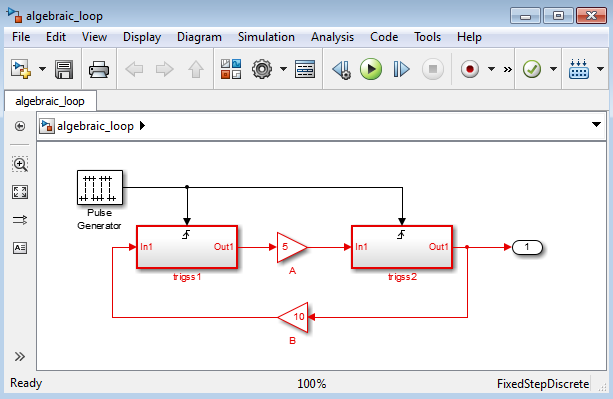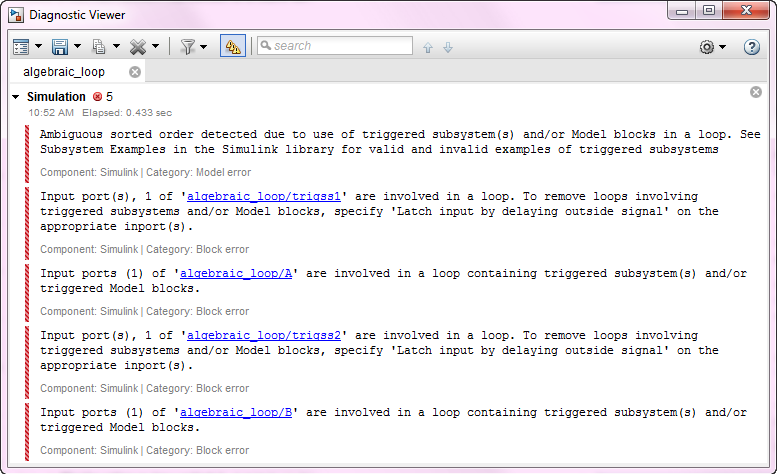## General Considerations when Building Simulink Models

### Avoiding Invalid Loops

You can connect the output of a block directly or indirectly (i.e., via other blocks) to its input, thereby, creating a loop. Loops can be very useful. For example, you can use loops to solve differential equations diagrammatically (see Model a Continuous System) or model feedback control systems. However, it is also possible to create loops that cannot be simulated. Common types of invalid loops include:

• Loops that create invalid function-call connections or an attempt to modify the input/output arguments of a function call (see Using Function-Call Subsystems for a description of function-call subsystems)

• Self-triggering subsystems and loops containing non-latched triggered subsystems (see Using Triggered Subsystems in the Using Simulink® documentation for a description of triggered subsystems and Inport in the Simulink reference documentation for a description of latched input)

• Loops containing action subsystems

For triggered and function-call subsystem examples, see Simulink Subsystem Semantics.

You might find it useful to study these examples to avoid creating invalid loops in your own models.

#### Detecting Invalid Loops

To detect whether your model contains invalid loops, select Update Model from the Modeling tab of the toolstrip. If the model contains invalid loops, the invalid loops are highlighted. This is illustrated in the following model.and displays an error message in the Diagnostic Viewer.If there are two Model files with the same name (e.g. `mylibrary.slx`) on the MATLAB® path, the one higher on the path is loaded, and the one lower on the path is said to be "shadowed".

Tip

To help avoid problems with shadowed files, turn on the Simulink preference Do not load models that are shadowed on the MATLAB path. See Do not load models that are shadowed on the MATLAB path.

The rules Simulink software uses to find Model files are similar to those used by MATLAB software. See What Is the MATLAB Search Path?. However, there is an important difference between how Simulink block diagrams and MATLAB functions are handled: a loaded block diagram takes precedence over any unloaded ones, regardless of its position on the MATLAB path. This is done for performance reasons, as part of the Simulink software's incremental loading methodology.

The precedence of a loaded block diagram over any others can have important implications, particularly since a block diagram can be loaded without the corresponding Simulink window being visible.

#### Making Sure the Correct Block Diagram Is Loaded

When using libraries and referenced models, you can load a block diagram without showing its window. If the MATLAB path or the current MATLAB folder changes while block diagrams are in memory, these block diagrams can interfere with the use of other files of the same name.

For example, open a model with a library called `mylib`, change to another folder, and then open another model with a library also called `mylib`. When you run the first model, it uses the library associated with the second model.

This can lead to problems including:

• Simulation errors

• Wrong results

#### Detecting and Fixing Problems

To help avoid problems with shadowed files, you can turn on the Simulink preference Do not load models that are shadowed on the MATLAB path. See Do not load models that are shadowed on the MATLAB path.

When updating a block diagram, the Simulink software checks the position of its file on the MATLAB path and will issue a warning if it detects that another file of the same name exists and is higher on the MATLAB path. The warning reads:

```The file containing block diagram 'mylibrary' is shadowed by a file of the same name higher on the MATLAB path.```
This may indicate that the wrong file called `mylibrary.slx` is being used. To see which file called `mylibrary.slx` is loaded into memory, enter:

``` which mylibrary C:\work\Model1\mylibrary.slx ```
To see all the files called `mylibrary` which are on the MATLAB path, including MATLAB scripts, enter:

```which -all mylibrary C:\work\Model1\mylibrary.slx C:\work\Model2\mylibrary.slx % Shadowed ```
To close the block diagram called `mylibrary` and let the Simulink software load the file which is highest on the MATLAB path, enter:
`close_system('mylibrary')`

### Model Building Tips

Here are some model-building hints you might find useful:

• Memory issues

In general, more memory will increase performance.

• Using hierarchy

More complex models often benefit from adding the hierarchy of subsystems to the model. Grouping blocks simplifies the top level of the model and can make it easier to read and understand the model. For more information, see Create Subsystems. The Model Browser provides useful information about complex models (see Simulink Editor).

• Cleaning up models

Well organized and documented models are easier to read and understand. Signal labels and model annotations can help describe what is happening in a model. For more information, see Signal Names and Labels and Annotate Models.

• Modeling strategies

If several of your models use the same blocks, you can save these blocks for easy reuse. For example, you can save a collection of blocks in a custom library. Then, when you build new models, you can copy these blocks from the library.

Generally, when building a model, design it first on paper, then build it using the computer. Then, when you start putting the blocks together into a model, add the blocks to the model window before adding the lines that connect them. This way, you can reduce how often you need to open block libraries.